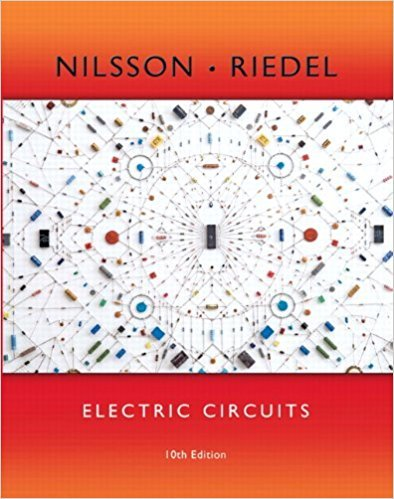×
×

# Solutions for Chapter 17.2: The Convergence of the Fourier Integral## Full solutions for Electric Circuits | 10th Edition

ISBN: 9780133760033Solutions for Chapter 17.2: The Convergence of the Fourier Integral

Solutions for Chapter 17.2
4 5 0 324 Reviews
30
4
##### ISBN: 9780133760033

Chapter 17.2: The Convergence of the Fourier Integral includes 2 full step-by-step solutions. This expansive textbook survival guide covers the following chapters and their solutions. Since 2 problems in chapter 17.2: The Convergence of the Fourier Integral have been answered, more than 13457 students have viewed full step-by-step solutions from this chapter. This textbook survival guide was created for the textbook: Electric Circuits, edition: 10. Electric Circuits was written by and is associated to the ISBN: 9780133760033.

Key Engineering and Tech Terms and definitions covered in this textbook
×